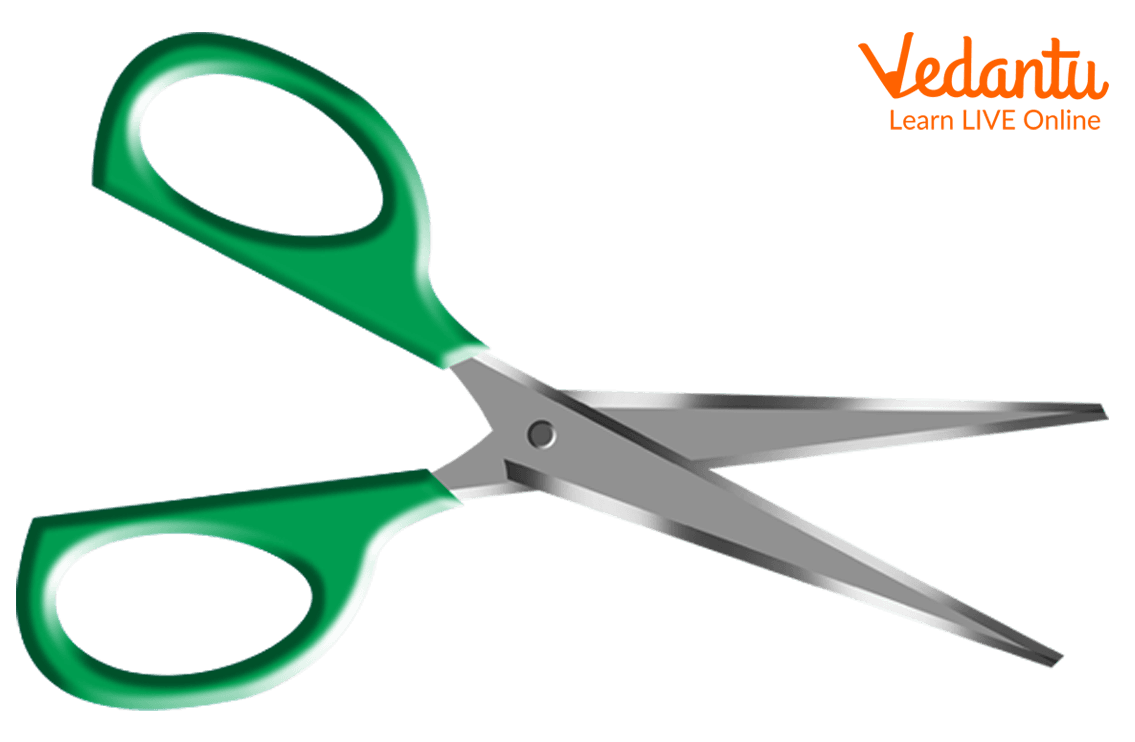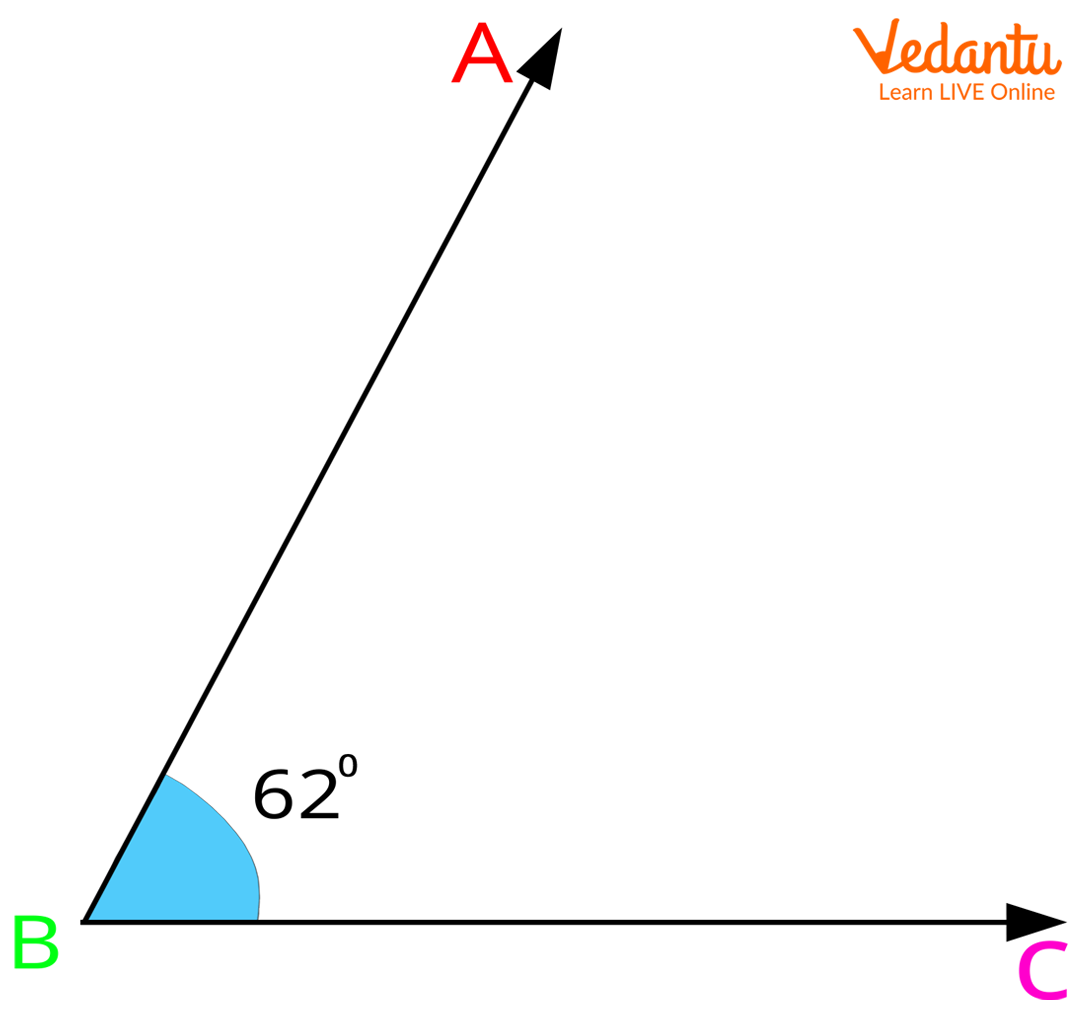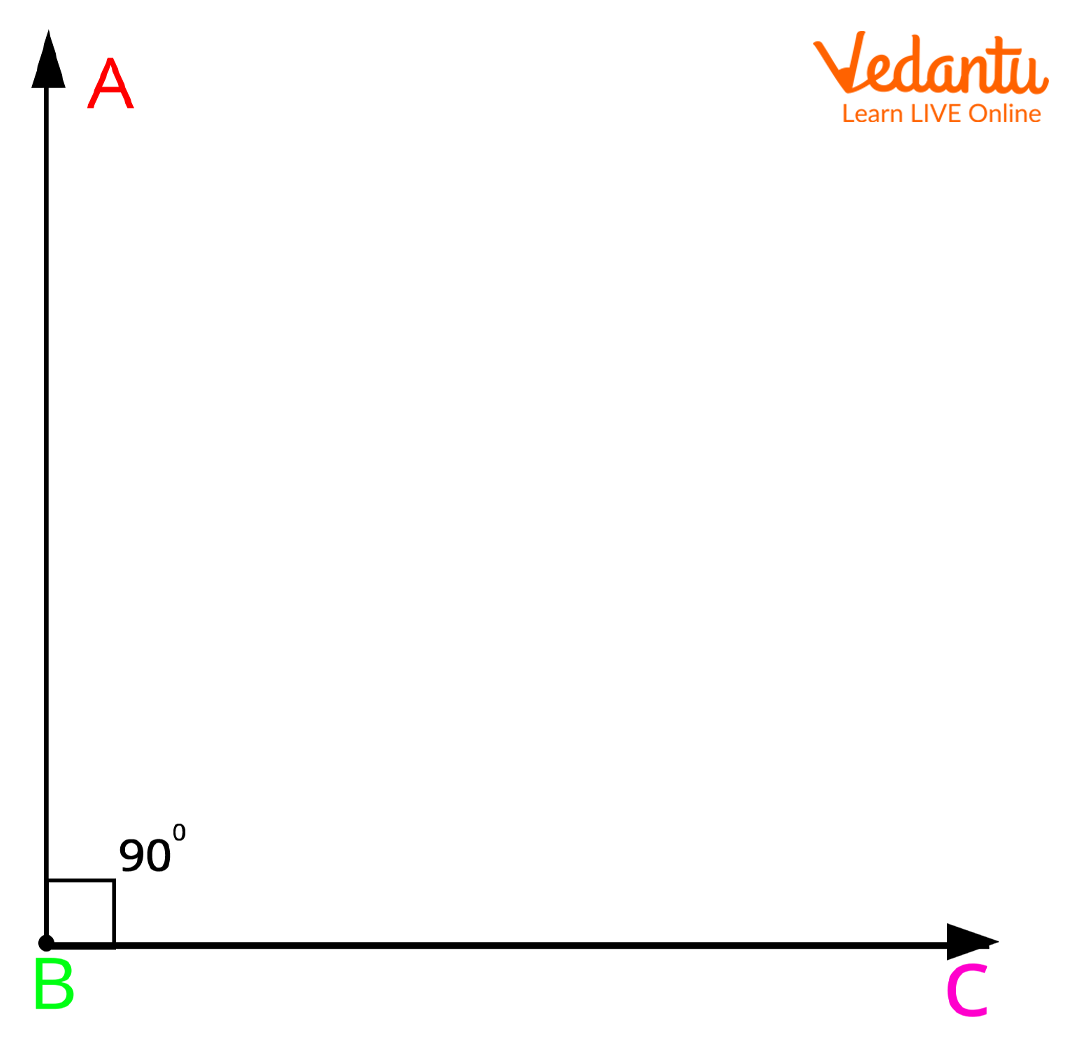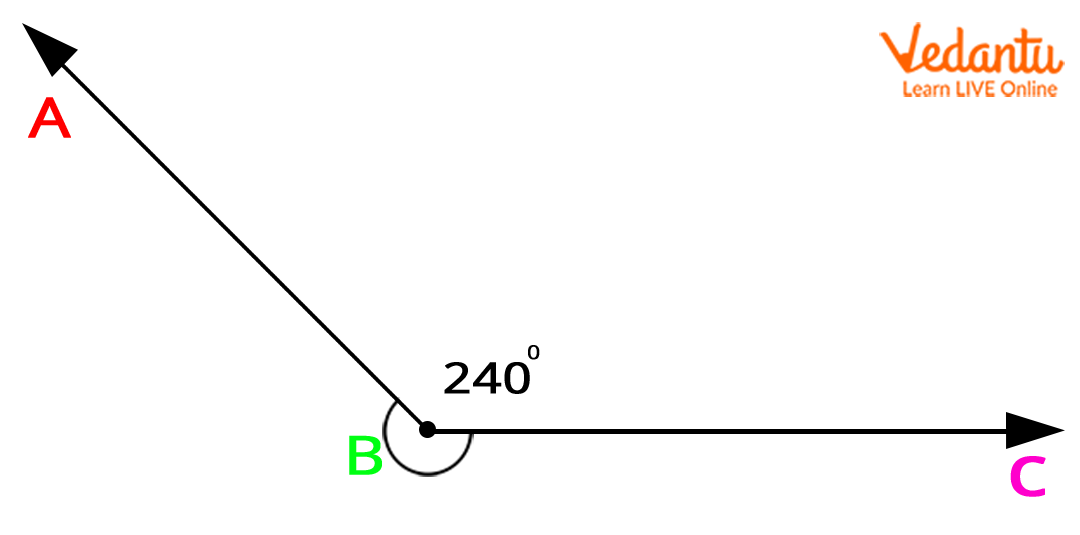Courses
Courses for Kids
Free study material
Free LIVE classes
More

# Angles SymbolLIVE
Join Vedantu’s FREE Mastercalss

You must have heard your elders say, “90 degrees angle” or “45 degrees angle” and every time you asked them what is the meaning of it, they must have told you, “You won’t understand. You are a kid”. Don’t worry! We are here to help you to be a smart kid. Let’s learn about angles and the angle symbol. Also, we have a fun activity and a quiz for you to make sure that next time an elder talks about angles, you know it already.

## Let’s Define an Angle

When two straight lines or rays meet at a common end-point, the shape formed is called an angle in geometry. The symbol ∠ shows what an angle looks like. Usually, angles are also represented by alphabets, such as ∠AOB or ∠ABC. Note that the middle alphabet, i.e., O in ∠AOB and B in ∠ABC are the common end points where the two lines meet to form the angle symbol.Image: Angle AOB

### Parts of an Angle

Now that you know what an angle is. Let’s learn about the parts of an angle.

Arms: The arms of an angle are also known as its sides. The two lines or rays that come together to form an angle are the arms or sides of an angle. For instance, in the image given below, side AB and side BC are the arms of ABC.Image: Sides AB and BC of angle ABC

Vertex: In ABC given above, side AB and BC have a common end point, which is point B. It is the point where the two arms of angle ABC meet. This point is called the vertex (plural: vertices) of an angle. Here, point B is the vertex of angle ABC.

### How to Measure an Angle?

Ask your brother or sister to show you a protractor from their geometry box. A protractor is an instrument used to measure an angle. Like all other objects angles too have units of measure. We are going to discuss the two main units that are used to measure the size of an angle, i.e., degree and radian.

Degree: It is the most standard unit to measure an angle. The degree symbol is represented by °. So, an angle of 60 degrees would be written as 60°. Furthermore, a degree can also be divided into minutes and seconds. One degree is equal to 60 minutes (1° = 60') and one minute is equal to 60 seconds (1' = 60").

Radian: This is another important unit of measure. Angles can also be measured and represented in radians that is by using pi (π). Hence, an angle measuring 180 degrees is equal to π in radians. So, 360 degrees would be the same as 2π in radians.

### Fun Activity!

As promised, we have a little fun activity for you since you are a few more steps away from being a pro in angles. There are four objects in the table below. Choose the one/s that have an angle/angles.Image: A pair of scissorsImage: A ballImage: A slice of pizzaImage: An open book

Look around your house. Can you find more such objects that have angles? Draw them in your notebook.

### Types of Angles

Now that we have learnt about the definition and parts of an angle and know how to measure it, let’s take a look at some of the types of angles. When classifying angles, their measurement is the deciding factor. It determines the type of angle. Let us discuss the six major types of angles in Geometry.

• Acute angle: An acute angle is greater than 0° but less than 90°.

• Obtuse angle: Any angle that is greater than 90° but less than 180° is considered an obtuse angle.

• Right angle: The right angle is the angle that measures 90 degrees.

• Straight angle: The angle measuring 180 degrees is known as the straight angle.

• Reflex angle: A reflex angle should be greater than 180° but less than 360°.

• Complete angle: An angle that forms a full circle and measures 360° is known as a complete angle.

 Name of Angle Trick to Remember Example Acute angle < 90°Image: Acute angle Obtuse angle > 90°Image: Obtuse angle Right angle = 90°Image: Right angle Straight angle =180°Image: Straight angle Reflex angle >180°Image: Reflex angle Complete angle =360°Image: Complete angle

### 2 Bonus Angles!

Here are two more types of angles for you. These include the positive angle and the negative angle.

• A positive angle is an angle measured in the anti-clockwise or counterclockwise direction.

• A negative angle, on the other hand, is the one that is measured in the clockwise direction.Image: Positive angle and negative angle

### Quiz Hour

Learning in itself is incomplete unless we absorb and retain the information we have gathered. Now that we are almost towards the end of the topic, let us revise all that we have learnt about angles through this short and easy quiz. Given below are five questions and three options for each question. Choose the correct answer from what you can recall.

Q1. Which of the following is the angle symbol?

1. ∠ (b) ° (c) π

Q2. The common end point where the arms of an angle meet is the______.

1. radian (b) vertex (c) protractor

Q3. If you have to measure the size of an angle, which of the following units would you use?

1. kilograms (b) seconds (c) degrees

Q4. A straight angle measures 180° and a complete angle measures 360°. Is the statement true?

1. true (b) false (c) partly true

Q5. A positive angle is measured in _____ direction and a negative angle in ____ direction.

1. clockwise, anti-clockwise (b) anti-clockwise, clockwise (c) clockwise, clockwise

In case you couldn’t remember a few answers, here are all the correct options. Check how many you got correct.

Q1. (a) ∠

Q2. (b) vertex

Q3. (c) degrees

Q4. (a) true

Q5. (b) anti-clockwise, clockwise

 Guess what!The word ‘angle’ has been taken from the Latin word ‘Angulus’.Eudemus was the first to come up with the concept of angles.An angle of zero degrees (0°) is called a zero angle.

### Conclusion

So we hope you have gathered a clear picture of what an angle is, the angle symbol, the various parts of an angle, the measurement of angles and the different types of angles. And we hope you had fun too! To learn more about such interesting concepts, head to our website and explore the different articles and worksheets we have prepared for you.

Last updated date: 24th Sep 2023
Total views: 137.7k
Views today: 3.37k

## FAQs on Angles Symbol

1. What are the six major classifications of angles?

Angles are mainly classified into six types based on their measurement. These include acute angle, obtuse angle, right angle, straight angle, reflex angle and complete angle. Acute angles measure less than 90 degrees; obtuse angles lie within the range of 90 to 180 degrees; right angles are of exact 90 degrees and straight angles of exact 180 degrees. A reflex angle could measure somewhere between 180 to 360 degrees whereas a complete circle is of 360 degrees. Based on directions, there are two types of angles. A positive angle is measured in the counterclockwise direction and a negative angle in the clockwise direction.

2. Why do we need angles?

Angle is a basic geometric figure. It has several important uses and purposes in our daily lives. Engineers and architects who construct roads, buildings and bridges need angles for correct measurements and designs. It is with the help of angles that they provide us with wonderful building structures. Artists use angles to paint pictures and collages. Photographers create magic by capturing images from the correct angles. Athletes use angles to determine and enhance their efficiency and performance. Carpenters use angles to carve out tables, chairs, cupboards and showpieces.